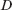hydrodynamic radius otherwise Stokes radius; Stokes–Einstein radius (rus. гидродинамический радиус) — size of the object, which is calculated on the assumption of its spherical shape using the value of diffusion coefficient in liquid.

### Description

In accordance with the Stokes-Einstein equation the diffusion coefficientof a sphere in liquid is equal to,

whereis the Boltzmann factor,is the temperature in degrees Kelvin,is fluid viscosity, andis the ball radius. The radius calculated as per the equation above using the diffusion coefficient value is called the hydrodynamic radius of a particle or a macromolecule.

In turn, the method based on inelastic scattering can be used to calculate the diffusion coefficient of colloidal particles in a solution. In some cases the accuracy of this method allows one to determine the formation of molecular adsorption layers on the surface of the particles from the change of their average hydrodynamic radius. Analysis of inelastic scattering data enables the calculation of the particle size distribution in a range from several nanometres to several micrometres.

#### Authors

• Khokhlov Alexey R.
• Govorun Elena N.

#### Source

1. Khokhlov A. R., Kuchanov S.I. Lectures on Physical Chemistry of Polymers (in Russian). — Moscow.: Mir, 2000. — 192 p.